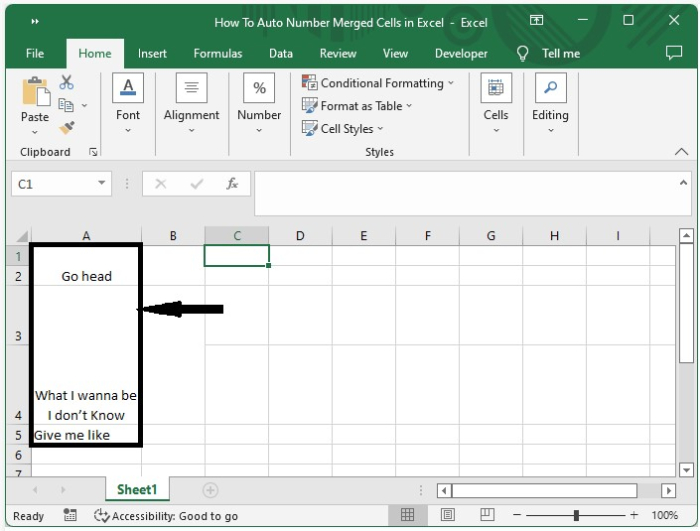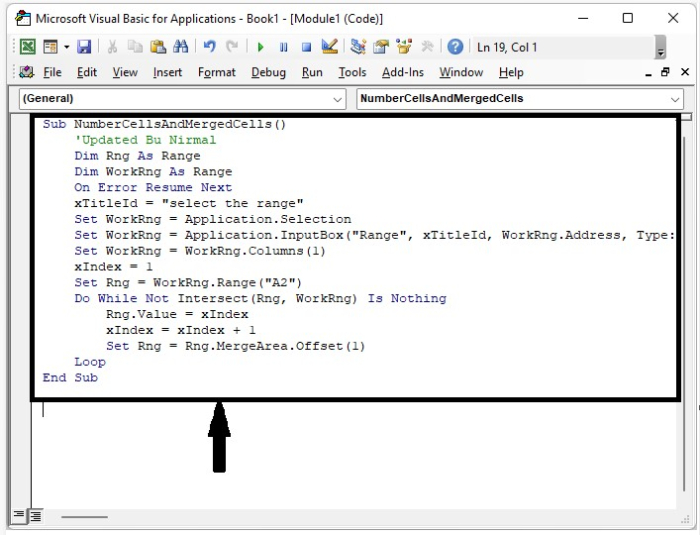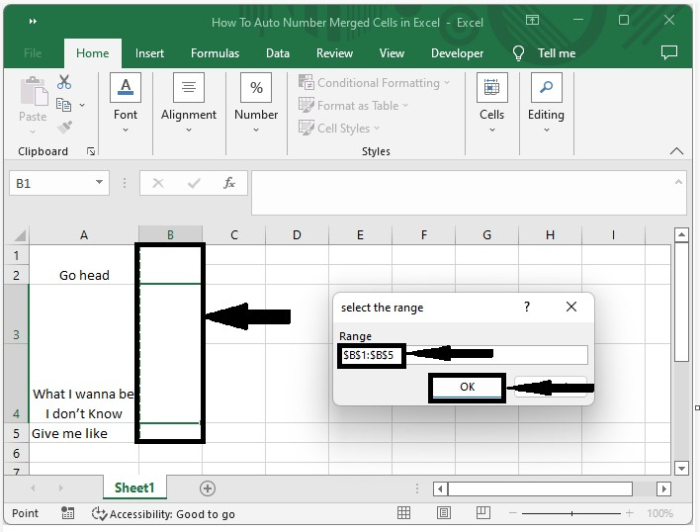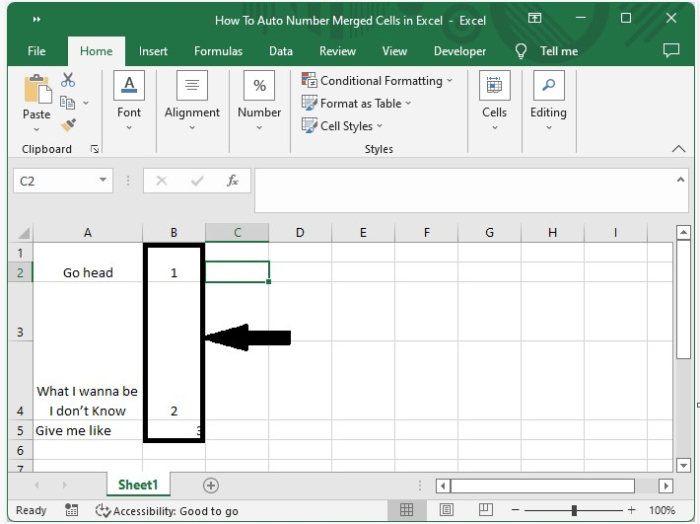# How to Auto-Number Merged Cells in Excel?

When we try to insert a sequence number into an item in Excel, we always use the autofill handle, but when we try to apply the same process to cells of different sizes, the error appears. This process can’t be completed using the default functions in Excel; instead, we will be using the VBA application to complete our process.

Read this tutorial to learn how you can autonumber merged cells in Excel.

## AutoNumber Merged Cells in Excel

Here, we will first insert a VBA module and then run the module to complete our task. Let us see a simple process to understand how we can auto-number merged cells in Excel.

Step 1

Let us consider an Excel sheet that contains a list of different heights, and then try to apply the sequence number using the autofill handle.We can see that the result is not accurate, but we can use VBA code to get the perfect result. Now right-click on the sheet name and select view code to open the vba application, and click on inset and select module, then type the programme into the text box as shown in the below image.

### Example

Sub NumberCellsAndMergedCells()
'Updated Bu Nirmal
Dim Rng As Range
Dim WorkRng As Range
On Error Resume Next
xTitleId = "select the range"
Set WorkRng = Application.Selection
Set WorkRng = Application.InputBox("Range", xTitleId, WorkRng.Address, Type:=8)
Set WorkRng = WorkRng.Columns(1)
xIndex = 1
Set Rng = WorkRng.Range("A2")
Do While Not Intersect(Rng, WorkRng) Is Nothing
Rng.Value = xIndex
xIndex = xIndex + 1
Set Rng = Rng.MergeArea.Offset(1)
Loop
End SubStep 2

Now save the workbook as a macro-enabled template and press F5 to run the code. A pop-up window titled "Select the Range" will appear; select the range of cells to number as shown in the image below.Step 3

Now click on OK to get our final output, as shown in the below image.## Conclusion

In this tutorial, we used a simple example to demonstrate how we can auto-number merged cells in Excel to highlight a particular set of data.

Updated on: 11-Jan-2023

511 Views